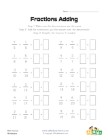# Search

About 245 Search Results Matching Types of Worksheet, Worksheet Section, Generator, Generator Section, Subjects matching Math, Grades matching 3rd Grade, Similar to Spring Beginning Letters Worksheet, Page 2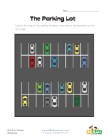## Parking Lot Story Problems Worksheet

Use the map of the cars in the parking lot to answ...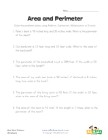## Area and Perimeter Word Problems Worksheet

Solve the 6 area and perimeter word problems in th...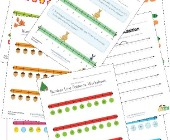## Number Line Worksheets

Check out our collection of number line worksheets...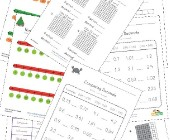## Decimal Worksheets

This collection of free decimal worksheets will he...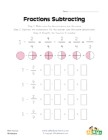## Simple Fraction Subtraction Worksheet 3

A slightly more involved, but still simple, fracti...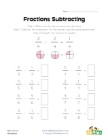## Simple Fraction Subtraction Worksheet 1

This fractions worksheet has 8 simple fraction sub...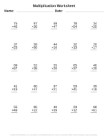## Multiplication Worksheet Generator

Generate printable multiplication worksheets using...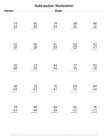## Subtraction Worksheet Generator

Generate printable subtraction worksheets using ou...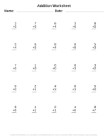Generate printable addition worksheets using our e...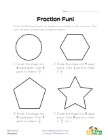## Fraction Fun Worksheet 2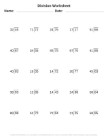## Division Worksheet Generator

Generate printable division worksheets using our e...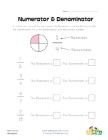## Numerator and Denominator Worksheet

Simple worksheet to work on identifying the numera...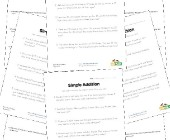## Word Problem Worksheets

Help children develop their problem solving skills...